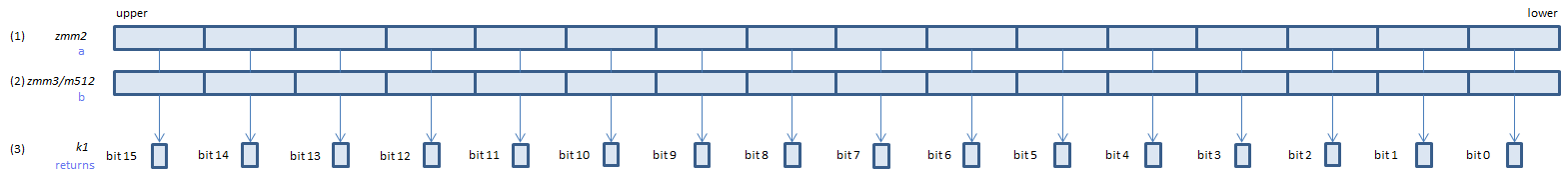﻿ vcmpeq_osps

## VCMPEQ_OSPS - CoMPare EQaul Ordered Signal Packed Single

VCMPEQ_OSPS xmm1, xmm2, xmm3/m128    (V1
__m128 _mm_cmp_ps(__m128 a, __m128 b, _CMP_EQ_OS)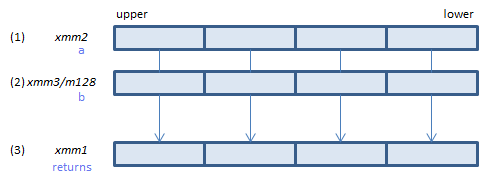For each float, if (1) == (2) set 1, else set 0, to all bits of the corresponding float of (3).
VCMPEQ_OSPS ymm1, ymm2, ymm3/m256    (V1
__m256 _mm256_cmp_ps(__m256 a, __m256 b, _CMP_EQ_OS)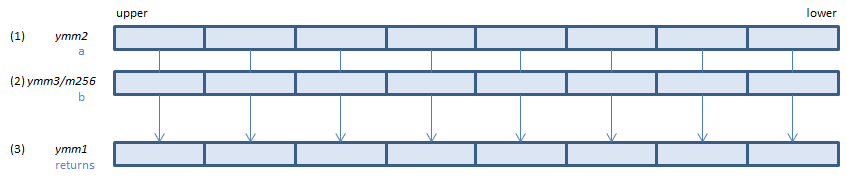For each float, if (1) == (2) set 1, else set 0, to all bits of the corresponding float of (3).
VCMPEQ_OSPS k1{k2}, xmm2, xmm3/m128/m32bcst    (V5+VL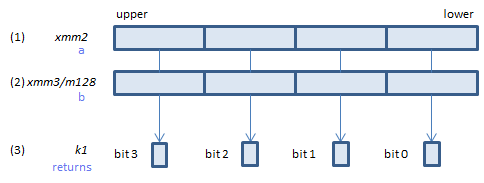For each float, if (1) == (2) set 1, else set 0, to the corresponding bit of (3).
If k2 bit is 0, the comparison is not done and the corresponding bit of (3) is set to zero. Upper bits of (3) are zero cleared.
VCMPEQ_OSPS k1{k2}, ymm2, ymm3/m256/m32bcst    (V5+VL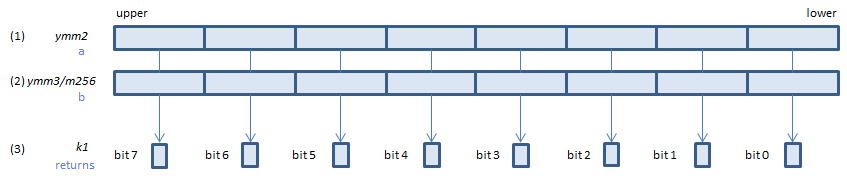For each float, if (1) == (2) set 1, else set 0, to the corresponding bit of (3).
If k2 bit is 0, the comparison is not done and the corresponding bit of (3) is set to zero. Upper bits of (3) are zero cleared.
VCMPEQ_OSPS k1{k2}, zmm2, zmm3/m512/m32bcst{sae}    (V5